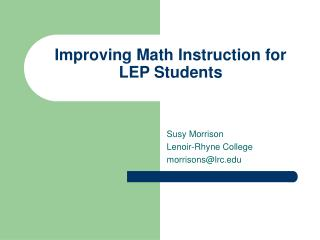Download PresentationImproving Math Instruction for LEP Students

# Improving Math Instruction for LEP Students

Download Presentation## Improving Math Instruction for LEP Students

- - - - - - - - - - - - - - - - - - - - - - - - - - - E N D - - - - - - - - - - - - - - - - - - - - - - - - - - -
##### Presentation Transcript

1. Improving Math Instruction for LEP Students Susy Morrison Lenoir-Rhyne College morrisons@lrc.edu

2. Purpose • Learn strategies to improve math instruction • Determine what changes would help all systems meet AYP • Discuss ways to collaborate with content teachers • Learn from each other

3. Questions to Ponder • What are the 5 strands of math on the SCS? • What vocabulary is essential to instruction? • Are manipulatives used in all classrooms? • What should a typical math lesson include? • Do your classroom teachers welcome inclusion? • How can teachers teach to their strength? • How do we get everyone on board? (teachers, principal, and students)

4. Quote Acquiring good math skills resembles building a skyscraper in that regardless of how high you go, you must first begin with a good foundation. Senitha Frost

5. Mathematics K-8 • 5 strands interwoven -Numbers & Operations -Measurement -Geometry -Data Analysis & Probability -Algebra • Concrete,pictorial, and symbolic representation • Problem-solving strategies

6. Mathematics 9-12 • 4 strands interwoven -Numbers & Operations -Geometry & Measurement -Data Analysis & Probability -Algebra

7. Introductory Math Geometry Algebra I Technical Math I Technical Math II Advanced Function Discrete Math Precalculus Integrated Math I Integrated Math II Integrated Math III Integrated Math IV AP Statistics AP Calculus High School Math Classes

8. Kindergarten-8th K-2 Math assessments Tested annually High School Need 3 math credits Algebra I (Level III) Grade Level Requirements

9. Group Activity • What are your math weaknesses? • Is math taught in your ESL classes? • Do your ESL teachers know what their students are doing in math? • What math programs are being used? • How is math assessed? • How often is math assessed?

10. Evaluation of Your Math Program • Visit schools, classes, and teachers • Frequently evaluate • Determine what is working well • Don’t be afraid to change • Support your changes

11. Checklist for Observing • ___discussing content & language objectives • ___building on prior knowledge • ___using supplementary material • ___engaging meaningful activities • ___stressing key vocabulary • ___employing a variety of techniques (manipulatives) • ___scaffolding • ___emphasizing HOT skills • ___providing wait time for student responses • ___listening, speaking, reading, and writing • ___using appropriate assessment

12. Barriers English Language Learners Face • Multiple meanings of words • Structures • Cultural knowledge • Decimals, commas, and periods • Square root/ division • Similar sounds of words

13. Math words with multiple meanings • operation • power • problem • plot • table • area • face • plane • tree

14. Math Structures • Add to… • Decrease by • Take away from • Greater than • Less than • 6 is 2 greater than 4 • If x = y, then… • Prepositions in word problems often signal operations • Passive voice (twenty is divided by five)

15. Addition add plus and combine sum total of more than increased by greater than Subtraction subtract minus less less than fewer decreased by difference lower take away Key Focus Words

16. multiply times product as a factor twice double triple groups of divide divided by quotient separated into equal groups shared equally over into how many groups Multiplication & Division

17. Cultural Knowledge • Different computational methods • U.S. monetary system • U.S. customary system/metric system • Writing numbers from left to right • Dates • Periods instead of commas • Commas instead of decimals • Similar sound of words • Square root/division

18. Make Math More Comprehensible • Increase the use of visuals • Integrate TPR • Use repetition • Use demonstrations • Use drama • Be aware of cultural differences • Increase the use of manipulatives

19. Word Problems • Highly language dependent • Must learn to write their own word problems • Learn key words to determine operation Lu has 3 plates of cupcakes. There are four cupcakes on each plate. How many cupcakes does Lu have altogether?

20. Manipulatives

21. Mathematical Terms A Glossary of Mathematical Terms for parents and teachers is available in a Spanish-English edition. http://community.learnnc.org/dpi/math/archives/GlossarySP.pdf

22. Explicit vocabulary Language & content objectives Less is more Technology Prefixes & suffixes Think aloud Predictable routines Think, pair, share Wait time Use consistent formats for test & assignments Word walls Math journals Chunking Manipulatives Real life problem solving Games to reinforce Repeat, repeat, repeat Effective Strategies

23. Recommendations • Share your expertise • Collaborate with math teachers • Integrate language and content objectives • Ask grade level teachers for key math vocabulary • Provide predictable classroom routines • Special scheduling accommodations must be made • Provide tutorials for struggling students • Follow through on goals and assessments